## Example Questions

### Example Question #1 : How To Find Permutation Notation

In permutation notation, what does 8P4 represent?

1680

6720

210

336

1680

Explanation:

The expression  8P4 represents the number of permutations of 8 objects arranged 4 at a time. Thus, 8 x 7 x 6 x 5 = 1680

### Example Question #11 : Permutation / Combination

How many different ways can five books be lined up on a shelf?

60

80

120

150

100

120

Explanation:

Order matters, so we use permutations:  (5)(4)(3)(2)(1) = 120

There are five possibilities for the first book, four possibilities for the second book, three for the third, and two for the fourth, and one possibility for the last book.

### Example Question #3 : How To Find Permutation Notation

How many different ways can cheese slices be stacked in piles containingunique types if you are presented with a selection ofdifferent cheeses?  (Presume that the order of the cheese slices does matter.)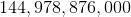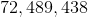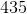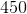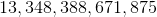Explanation:

Since the order matters, you are dealing with a permutation in this question. A permutation like this could be done with the equation: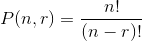For our values, this would be: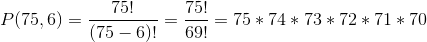However, it is easiest just to think of this like it hasslots. Into the first, you havechoices, into the second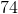, and so forth. This generates for you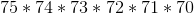more easily.

This is. That is a lot of cheese arrangements!